## 提升Python代码性能的六个技巧

article2023/12/12 3:44:33/

### 文章目录

• 前言
• 为什么要写本文？
• 1、代码性能检测
• 1.1、使用 timeit 库
• 1.2、使用 memory_profiler 库
• 1.3、使用 line_profiler 库
• 2、使用内置函数和库
• 3、使用内插字符串 f-string
• 4、使用列表推导式
• 5、使用 lru_cache 装饰器缓存数据
• 6、针对循环结构的优化
• 7、选择合适算法和数据结构
• 8、推荐书籍
• 结语

# 前言

🗿 hello大家好啊，我是作家桑。本文为大家介绍提升 Python 代码性能的六个技巧，希望大家看完有所收获。

# 为什么要写本文？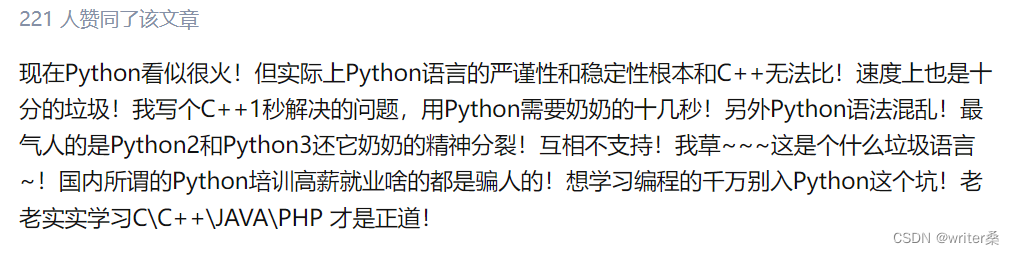# 1、代码性能检测

``````# 斐波那契数列
def Fibonacci():
a, b = 0, 1
i = 0
while i < 100:
print(b)
a, b = b, a+b
i += 1

Fibonacci()
``````

## 1.1、使用 timeit 库

timeit 模块是 Python 的内置模块。timeit 模块致力于衡量代码的性能，模块内提供了许多个函数和类，以便开发者能够精确地测量代码的执行时间。timeit 模块用法较为简单，适合用来计算一小段代码的运行时间。

``````import timeit

def Fibonacci():
a, b = 0, 1
i = 0
while i < 100:
print(b)
a, b = b, a+b
i += 1

result = timeit.timeit(Fibonacci, number=5)

print(f"Fibonacci函数的运行时间为: {result}")
``````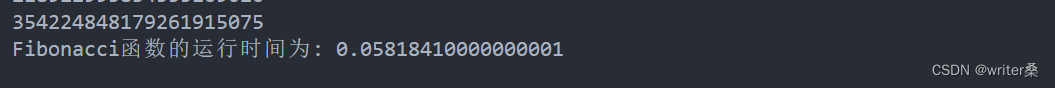## 1.2、使用 memory_profiler 库

memory_profiler 是 Python 的第三方库（需要使用 pip 命令进行安装），是一个可根据每行代码查看内存占用的工具。开发者使用 memory_profiler 库可以有效的定位到程序中占有内存最多的代码，以此找到程序运行的瓶颈。

pip 命令安装：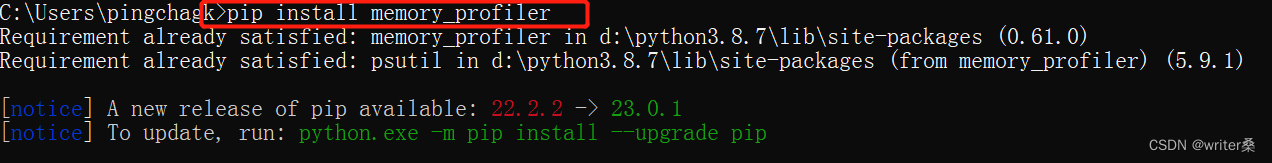``````from memory_profiler import profile

@profile
def Fibonacci():
a, b = 0, 1
i = 0
while i < 100:
print(b)
a, b = b, a+b
i += 1

Fibonacci()
``````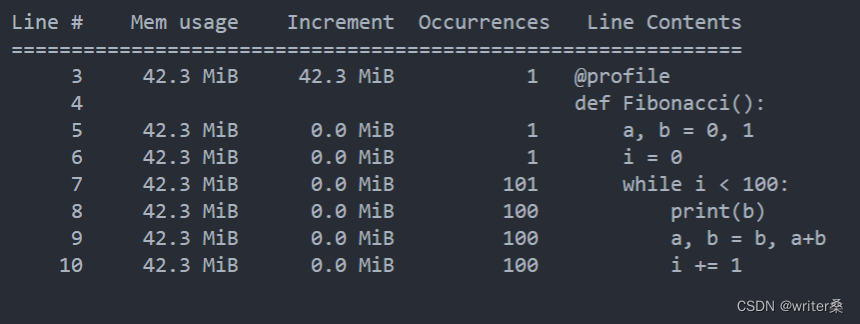## 1.3、使用 line_profiler 库

``````from line_profiler import LineProfiler

def Fibonacci():
a, b = 0, 1
i = 0
while i < 100:
print(b)
a, b = b, a+b
i += 1

lp = LineProfiler()
lp_wrap = lp(Fibonacci)
lp_wrap()

# 输出统计数据
lp.print_stats()
``````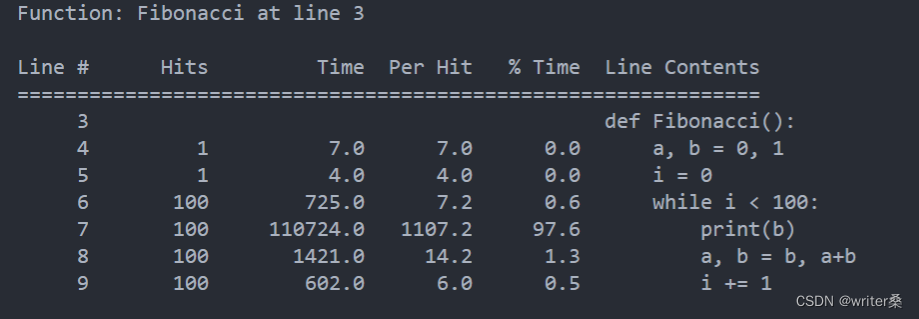# 2、使用内置函数和库

Python 的内置函数和库与我们常用的自定义函数、自定义数据类型相比，运行速度会显得非常快。这主要是因为内置数据类型的底层是使用 C 语言实现的，而 C 语言又是目前为止执行效率最高的高级语言，这是使用 Python 所无法比较的。而且 Python 的开发团队也对这些内置函数和库进行了良好的测试和优化。

``````my_list = []
word_list = "hello,world"

for word in word_list:
my_list.append(word.upper())

print(my_list)
``````

``````word_list = "hello,world"

# 使用内置map函数
my_list = map(str.upper, word_list)

print(list(my_list))
``````

# 3、使用内插字符串 f-string

``````places = 3
number = 1.23456

my_str = "number值和places值分别为{0}和{1}".format(number, places)

print(my_str)
``````

``````places = 3
number = 1.23456

my_str = f"number值和places值分别为{number}和{places}"

print(my_str)
``````

# 4、使用列表推导式

``````my_list = []

# 计算1到100以内的奇数
for i in range(1, 100):
if i % 2 == 1:
my_list.append(i)

print(my_list)
``````

``````my_list = [i for i in range(1, 100) if i % 2 == 1]

print(my_list)
``````

# 5、使用 lru_cache 装饰器缓存数据

``````import time

def my_func(x):
time.sleep(2)       # 模拟程序执行时间
return x

print(my_func(1))
print("=========")
print(my_func(1))
``````

``````import functools
import time

# 最多缓存128个不同的结果
@functools.lru_cache(maxsize=2)
def my_func(x):
time.sleep(2)       # 模拟程序执行时间
return x

print(my_func(1))
print("=========")
print(my_func(1))       # 结果已被缓存，无需等待立即返回
``````

# 6、针对循环结构的优化

``````my_list = []
word_list = ["hello,", "word"]

for word in word_list:
new_str = str.lower(word)        # 不必要的重复操作和点运算符
my_list.append(new_str)

print(my_list)
``````

``````my_list = []
word_list = ["hello,", "word"]
lower = str.lower

for word in word_list:
my_list.append(lower(word))

print(my_list)
``````

# 7、选择合适算法和数据结构

``````# 在有序数组中，使用二分查找算法查找元素要比使用顺序查找算法效率更高
def sequential_search(nums,target):
for num in nums:
if num == target:
return nums.index(num)
return -1			# 返回-1表示没有找到目标元素

nums = [1, 8, 10, 11, 22]
target = 11
print(sequential_search(nums, target))
``````

``````# 二分查找
def binary_search(nums,target):
first,last = 0, len(nums) - 1           # 定义数组的第一个元素下标和最后一个元素下标

while first <= last:                    #左闭右闭区间
mid_index = (first + last) // 2     #中间元素的下标值

if nums[mid_index] < target:
first = mid_index + 1
elif nums[mid_index] > target:
last = mid_index - 1
else:
return mid_index
return -1

nums = [1, 8, 10, 11, 22]
target = 11
print(binary_search(nums, target))
``````

# 8、推荐书籍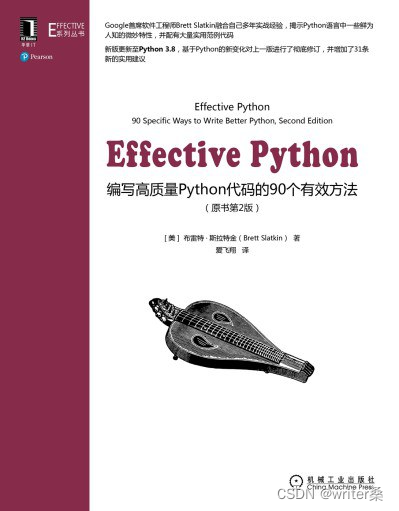# 结语

🎪 以上就是提升 Python 代码性能的技巧介绍啦，希望对大家有所帮助。感谢大家的支持。

### React项目规范：目录结构、根目录别名、CSS重置、路由、redux、二次封装axios

React项目&#xff08;一&#xff09;一、创建项目二、目录结构三、craco配置别名并安装less1.craco安装2.配置别名3.安装less四、CSS样式重置五、配置路由六、配置Redux1.创建大仓库2.创建小仓库&#xff08;1&#xff09;方式1&#xff1a;RTK&#xff08;2&#xff09;方式2…

### toString()、equals()是什么，为啥需要重写，多种方法来重写

https://m.runoob.com/java/java-object-class.html toString() 1.为什么会有toString 子类继承父类就可以使用父类所有非私有的属性的方法。 在Java中所有类都直接或者间接继承Object类&#xff0c;可以说只要是Object类里面定义的非私有的属性和方法&#xff0c;任何类都可…

### 【动态规划】不同路径，编辑距离题解及代码实现

Halo&#xff0c;这里是Ppeua。平时主要更新C语言&#xff0c;C&#xff0c;数据结构算法......感兴趣就关注我吧&#xff01;你定不会失望。 &#x1f308;个人主页&#xff1a;主页链接 &#x1f308;算法专栏&#xff1a;专栏链接 我会一直往里填充内容哒&#xff01; &…

### CKA最新考试费用是多少？考试内容是什么？

CKA认证考试是由Linux基金会和云原生计算基金会(CNCF)创建的&#xff0c;以促进Kubernetes生态系统的持续发展。该考试是一种远程在线、有监考、基于实操的认证考试&#xff0c;需要在运行Kubernetes的命令行中解决多个任务。CKA认证考试是专为Kubernetes管理员、云管理员和其他…